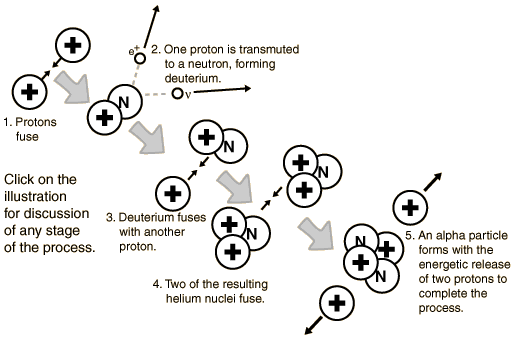## Wednesday, September 27, 2006

How dry this article in comparison to what we can witness in the cosmo?

One gets this sense of "curvature implied" that the events connected at locations in the universe could have been felt in other locations? What connects them? The spacetime fabric?:)

If one was speaking and one was seeing beyond the observation of the events shown here, then what is there to say if one cannot accept "the language" while they hold to only what they see? No speculation, no theories, no future?

Only, the now?With the development of quantum mechanics, it was realized that on this scale the protons must be considered to have wave properties and that there was the possibility of tunneling through the coulomb barrier

So while this information exist here now, how does this help you look at events, or is this information lost?

Virial Theorem

A general theorem from the mathematics of physics becomes a useful part of the picture of gravitational collapse. In the context of gravity it can be applied to a finite collection of particles which interact with each other by gravitational attraction. We can attribute to the collection of particles a total gravitational potential energy and a total kinetic energy. The virial theorem states that

Average kinetic energy = -1/2 x Average potential energy
One application of this theorem would be to a known mass of hydrogen gas in a proto-star. If you had a good estimate of the mass of the gas and could measure a sample of particle velocities to determine the kinetic energy, then you could predict the kinetic energy as the gas cloud underwent gravitational collapse. So for a given radius of collapse, you could make a prediction of the temperature of the hydrogen gas in terms of the kinetic energy and could make a prediction about when it would reach the ignition temperature for hydrogen fusion.

Another potential application is to the question of dark matter. If you examine a system of objects out in space and are able to measure the kinetic energy of the system, then you can imply the gravitational potential energy. If the total mass of all the visible objects is too small to give that amount of gravitational potential energy, then the implication is that there is matter there which you cannot see (dark matter). When this has been done for a variety of types of galaxies, there is strong evidence for dark matter (Baez).

Still the connection "is" in the "spacetime fabric" and all events have to be detailed according to some "relation of value in the energy distributed of itself," as we gaze upon the beautiful events within that cosmo?